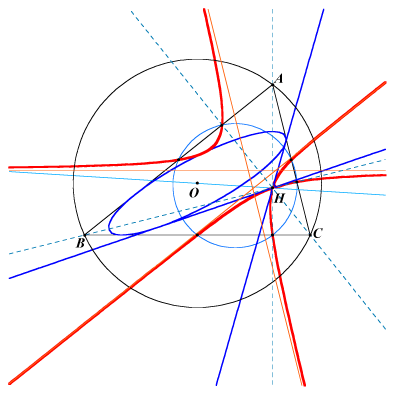see the general equation in CL010X(4), X(1650), X(57290), X(57291), X(57292), X(57293), X(57294), X(57295), X(57296) midpoints of ABC points at infinity of ABC sidelines vertices of the orthic triangleThe Allardice cubic A1(H) is the locus of the center of a circum-conic such that one of its asymptotes passes through H. The other asymptote envelopes the in-conic with center O, perspector X(69). The tangents at the node H to A1(H) are those drawn from H to this in-conic. K051 is a member of the class CL010 of cubics. K051 is the complement of cK(#X20, X2).